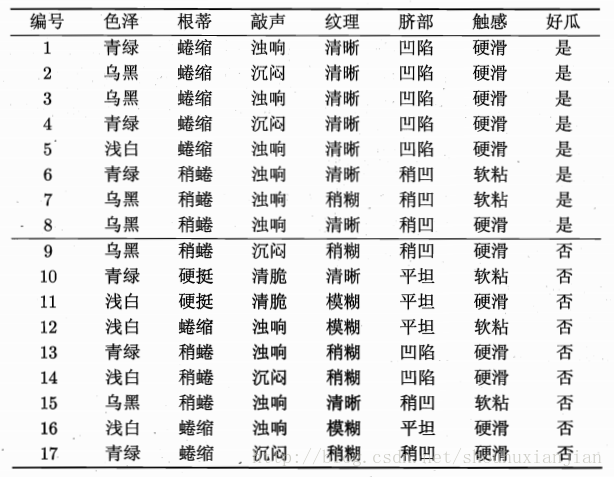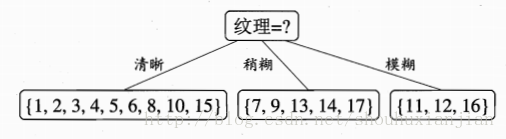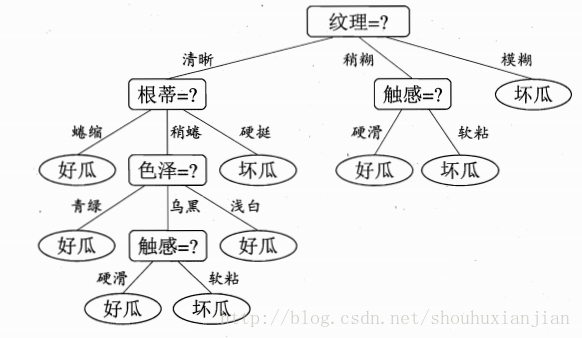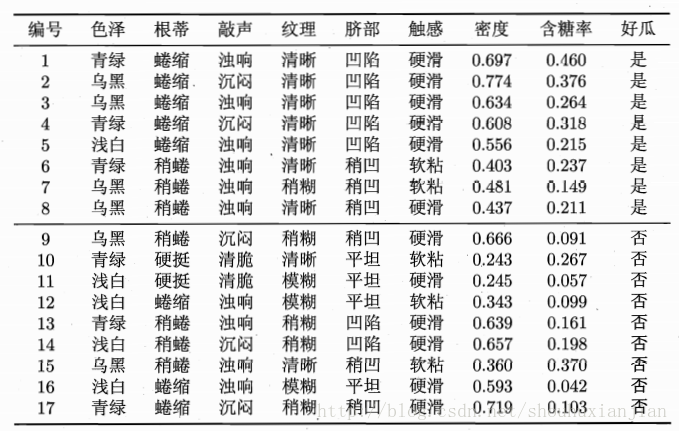# 决策树-笔记（1）

### 1 - 背景

ps：参数化模型，有着固定数量的参数，通常在预测阶段速度很快，而缺点就是对数据分布有着先验假设；非参数化模型，其参数会随着训练集的增大而增加，所以相对更加灵活，可是对于大数据集来说，其计算效率相对低下。

1）这是一棵空树；
2）这是一棵只有根节点的树；
3）这是一棵只有根节点，叶子节点的树；
4）这是一棵具有根节点、内部节点、叶子节点的完整树。

### 2 - 判别特征的选取规则

#### 2.1 - 信息增益：

$Ent(D) = -\sum_{k=1}^{|y|} p_klog_2p_k\tag{2.1}$

$|y|$表示样本的总类，如果$|y|=2$那么这就是个二分类数据集；$p_k$表示第$k$类样本在所有样本中占的比例为。且$Ent(D)$表示的就是所要划分的节点的信息熵，而接下来就是需要确定以哪个特征作为下一个判别特征从而划分该节点。假定当前可选的特征集合为$F$,其第$i$个特征为$F_i$。假定当前考虑的特征$F_i$一共有$V$种情况，那么如果以当前特征作为判别特征，则会从当前需要划分的节点上生成$V$条连接，那么这$V$条连接中，第$v$个连接上的样本个数，假定为$|D^v|$，那么第$v$个连接上的权重概率即为$\frac{|D^v|}{|D|}$，可以看出，权重越大，则当前连接划分的样本就越多。从而，计算出如果使用当前特征作为判别特征，那么其信息增益为：

$Gain(D,F_i) = Ent(D)-\sum_{v=1}^V\frac{|D^v|}{|D|}Ent(D^v) \tag{2.2}$

##### 2.1.1 - 离散特征1 - 根节点的确定

$Ent(D) = -\sum_{k=1}^2p_klog_2p_k=-(\frac{8}{17}log_2\frac{8}{17}+\frac{9}{17}log_2\frac{9}{17})=0.998\tag{2.3}$

$D^1$(色泽=青绿)={1，4，6，10，13，17}，且只有2种样本类别，则$p_1 = \frac{3}{6}$,$p_2=\frac{3}{6}$
$D^2$(色泽=乌黑)={2，3，7，8，9，15},则$p_1=\frac{4}{6}$,$p_2=\frac{2}{6}$
$D^3$(色泽=浅白)={5，11，12，14，16},则$p_1 = \frac{1}{5}$,$p_2=\frac{4}{5}$

$Ent(D^1) = 1.000$,$Ent(D^2)=0.918$,$Ent(D^3)=0.722$其信息增益为：

$Gain(D,色泽)= Ent(D)-\sum_{v=1}^3\frac{|D^v|}{|D|}Ent(D^v)=0.109 \tag{2.4}$

$Gain(D,根蒂)=0.143$$Gain(D,敲声)=0.141$$Gain(D,纹理)=0.381$$Gain(D,脐部)=0.289$$Gain(D,触感)=0.006$ps:因为是离散性质的特征，所以对于第二层来说，就可以直接将使用过的特征剔除当前层的候选判别特征集合了，因为是基于父节点的先验概率基础上做的进一步划分，即第二层只要基于{色泽、根蒂、敲声、脐部、触感}来考虑怎么划分，而如果是连续型的特征，就可以不做剔除这一步,只是缩小范围，后面会说到。
2 - 第二层节点的确定

1）先计算节点的信息熵：$Gain(D^1) =-(\frac{7}{9}log_2\frac{7}{9}+\frac{2}{9}log_2\frac{2}{9})=0.764 $2)$Gain(D^1,色泽) = 0.764-(\frac{4}{9}[-(\frac{3}{4}log_2\frac{3}{4}+\frac{1}{4}log_2\frac{1}{4})]+\frac{4}{9}[-(\frac{3}{4}log_2\frac{3}{4}+\frac{1}{4}log_2\frac{1}{4})]+\frac{1}{9}(\frac{1}{1}log_2\frac{1}{1}))=0.043$同理：$Gain(D^1,根蒂)=0.458$; $Gain(D^1,敲声)=0.331$; $Gain(D^1,脐部)=0.458$; $Gain(D^1,触感)=0.458$;

$D^1 = \{1,2,3,4,5\}$; $D^2=\{6,8,15\}$; $D^3 = \{10\}$##### 2.1.2 - 连续特征$\begin{eqnarray} Gain(D,F_i) &=& max_{t}Gain(D,F_i,t)\\ &=& max_{t}Ent(D)-\sum_{\lambda \in \{-,+\} }\frac{|D^\lambda|}{|D|}Ent(D_t^\lambda) \end{eqnarray} \tag{2.5}$

$\{0.244, 0.294, 0.351, 0.381, 0.420, 0.459, 0.518, 0.574, 0.600, 0.621, 0.636, 0.648, 0.661, 0.681, 0.708, 0.746\}$

$Gain(D,密度,0.381) = 0.998-(-[\frac{13}{17}(\frac{8}{13}log_2\frac{8}{13}+\frac{5}{13}log_2\frac{5}{13})+\frac{4}{17}(\frac{0}{4}log_2\frac{0}{4}+\frac{4}{4}log_2\frac{4}{4})]) = 0.262$

$Gain(D,色泽)=0.109$$Gain(D,根蒂)=0.143$$Gain(D,敲声) =0.141$$Gain(D,纹理)=0.381$$Gain(D,脐部) = 0.289$$Gain(D,触感) = 0.006$$Gain(D,密度) = 0.262$$Gain(D,含糖率)=0.349$

ps:假如选取了密度为当前的判别特征，则其左边子树是密度小于0.381的样本，右子树是大于0.381的点。因为该特征是连续值，故而不会被剔除下一层的判别特征的候选集合，因为对于小于0.381的样本集合(即左子树)来说，其还是可以从范围$[min,0.381)$接着进一步选取下一层的最佳划分点，然后将其离散化。

#### 2.2 - 信息增益率

$Gain\_ratio(D,F_i) = \frac{Gain(D,F_i)}{IV(F_i)} \tag{2.6}$

$IV(F_i) = -\sum_{v=1}^V\frac{|D^v|}{|D|}log_2\frac{|D^v|}{|D|} \tag{2.7}$

$IV(触感,V=2) =-(\frac{5}{17}log_2\frac{5}{17}+\frac{12}{17}log_2\frac{12}{17}) = 0.874$

$IV(色泽,V=3) = 1.580$

$IV(编号,V=17) = 4.088$

#### 2.3 - 基尼系数

$\begin{eqnarray}Gini(D) &=& \sum_{k=1}^{|y|} \sum_{k^ \neq k}p_kp_{k^}\\ &=& 1 - \sum_{k=1}^{|y|}p_k^2 \end{eqnarray}$

$Gini\_index(D,F_i) = \sum_{v=1}^V\frac{|D^v|}{|D|}Gini(D^v)$

2017/03/17 第一次修改！

 模式识别 第4版.(希腊)西奥多里蒂斯
 周志华，机器学习


posted @ 2018-10-11 16:10  仙守  阅读(883)  评论(0编辑  收藏  举报